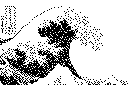We do the math so you don't have to!# Generating Gaussian Random Numbers

This note is about the topic of generating Gaussian pseudo-random numbers given a source of uniform pseudo-random numbers. This topic comes up more frequently than I would have expected, so I decided to write this up on one of the best ways to do this. At the end of this note there is a list of references in the literature that are relevant to this topic. You can see some code examples that implement the technique, and a step-by-step example for generating Weibull distributed random numbers.

There are many ways of solving this problem (see for example Rubinstein, 1981, for an extensive discussion of this topic) but we will only go into one important method here. If we have an equation that describes our desired distribution function, then it is possible to use some mathematical trickery based upon the fundamental transformation law of probabilities to obtain a transformation function for the distributions. This transformation takes random variables from one distribution as inputs and outputs random variables in a new distribution function. Probably the most important of these transformation functions is known as the Box-Muller (1958) transformation. It allows us to transform uniformly distributed random variables, to a new set of random variables with a Gaussian (or Normal) distribution.

The most basic form of the transformation looks like:

```         y1 = sqrt( - 2 ln(x1) ) cos( 2 pi x2 )
y2 = sqrt( - 2 ln(x1) ) sin( 2 pi x2 )
```
We start with two independent random numbers, x1 and x2, which come from a uniform distribution (in the range from 0 to 1). Then apply the above transformations to get two new independent random numbers which have a Gaussian distribution with zero mean and a standard deviation of one.

This particular form of the transformation has two problems with it,

1. It is slow because of many calls to the math library.
2. It can have numerical stability problems when x1 is very close to zero.
These are serious problems if you are doing stochastic modelling and generating millions of numbers.

The polar form of the Box-Muller transformation is both faster and more robust numerically. The algorithmic description of it is:

```         float x1, x2, w, y1, y2;

do {
x1 = 2.0 * ranf() - 1.0;
x2 = 2.0 * ranf() - 1.0;
w = x1 * x1 + x2 * x2;
} while ( w >= 1.0 );

w = sqrt( (-2.0 * ln( w ) ) / w );
y1 = x1 * w;
y2 = x2 * w;

```
where ranf() is the routine to obtain a random number uniformly distributed in [0,1]. The polar form is faster because it does the equivalent of the sine and cosine geometrically without a call to the trigonometric function library. But because of the possiblity of many calls to ranf(), the uniform random number generator should be fast (I generally recommend R250 for most applications).

Note: I wrote the preceeding paragraph more than 20 years ago and I was mostly concerned with the performance of embedded systems. With modern hardware you might find that the direct form is faster. You should benchmark the direct and polar forms in your target environment.

`  Probability transformations for Non Gaussian distributions `
Finding transformations like the Box-Muller is a tedious process, and in the case of empirical distributions it is not possible. When this happens, other (often approximate) methods must be resorted to. See the reference list below (in particular Rubinstein, 1981) for more information.

There are other very useful distributions for which these probability transforms have been worked out. Transformations for such distributions as the Erlang, exponential, hyperexponential, and the Weibull distribution can be found in the literature (see for example,MacDougall, 1987).

`                                           Useful References `
Box, G.E.P, M.E. Muller 1958; A note on the generation of random normal deviates, Annals Math. Stat, V. 29, pp. 610-611

Carter, E.F, 1994; The Generation and Application of Random Numbers , Forth Dimensions Vol XVI Nos 1 & 2, Forth Interest Group, Oakland California

Knuth, D.E., 1981; The Art of Computer Programming, Volume 2 Seminumerical Algorithms, Addison-Wesley, Reading Mass., 688 pages, ISBN 0-201-03822-6

MacDougall,M.H., 1987; Simulating Computer Systems, M.I.T. Press, Cambridge, Ma., 292 pages, ISBN 0-262-13229-X

Press, W.H., B.P. Flannery, S.A. Teukolsky, W.T. Vetterling, 1986; Numerical Recipes, The Art of Scientific Computing, Cambridge University Press, Cambridge, 818 pages, ISBN 0-512-30811-9

Rubinstein, R.Y., 1981; Simulation and the Monte Carlo method, John Wiley & Sons, ISBN 0-471-08917-6Dr. Everett (Skip) F. Carter Jr. Taygeta Scientific Inc. 1340 Munras Ave., Suite 314 Monterey, CA. 93940-6140 voice: 831.641.0645 FAX: 831.641.0647 INTERNET:skip@taygeta.com WWW:http://www.taygeta.com/Taygeta's home page